# Selina Solutions Concise Maths Class 10 Chapter 2 Banking (Recurring Deposit Accounts) Exercise 2(A)

A Recurring Deposit Account is abbreviated as R.D. Account. The maturity value of R.D. Account includes the amount deposited by the account holder together with interest compounded quarterly at a fixed rate. In this exercise, the students will solve problems based on this topic. The Selina Solutions for Class 10 Maths is the right place to find all the solutions to these problems. Students wishing to get a good grip over the concept can make use of the Selina Solutions Concise Maths Class 10 Chapter 2 Banking (Recurring Deposit Accounts) Exercise 2(A) PDF, which is available below.

## Selina Solutions Concise Maths Class 10 Chapter 2 Banking (Recurring Deposit Accounts) Exercise 2(A) Download PDF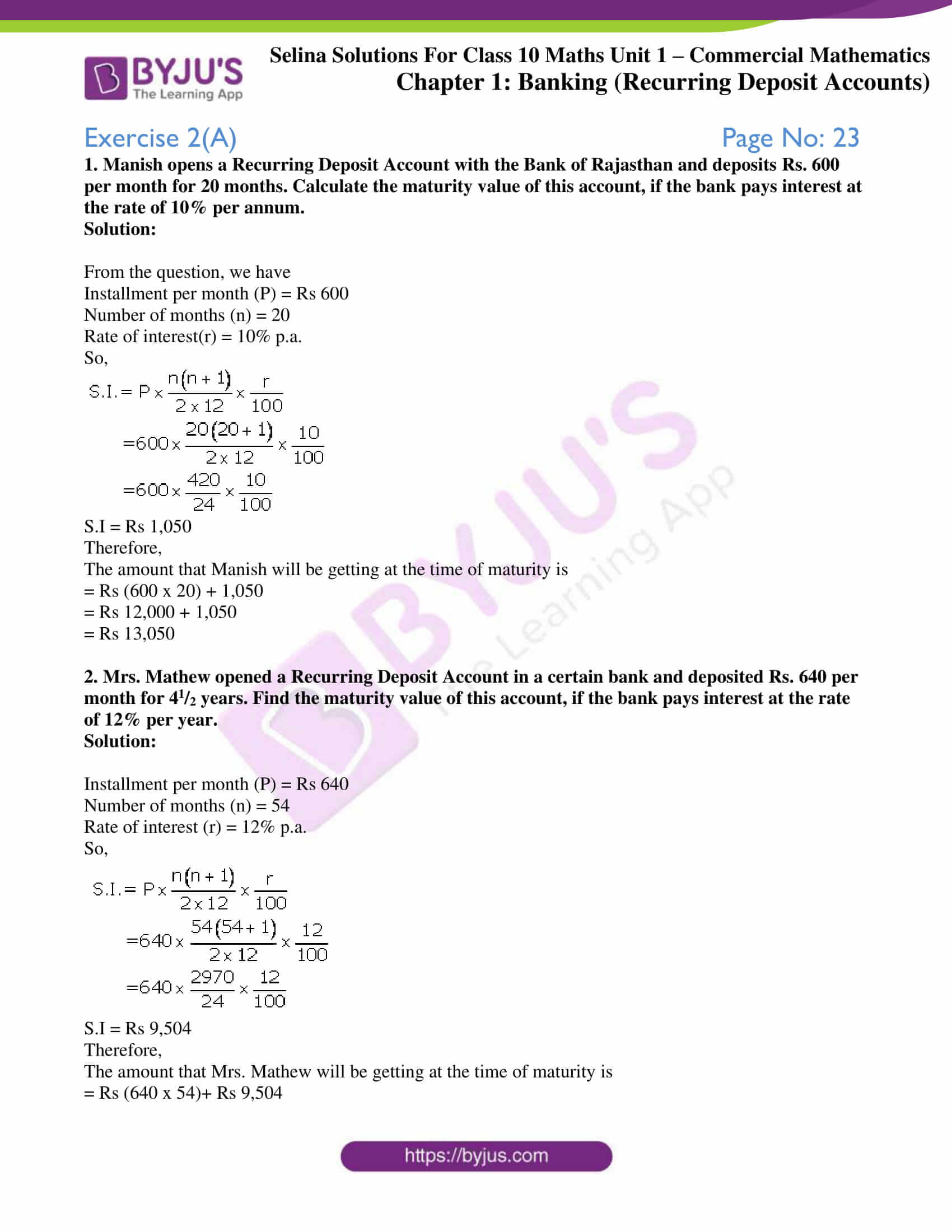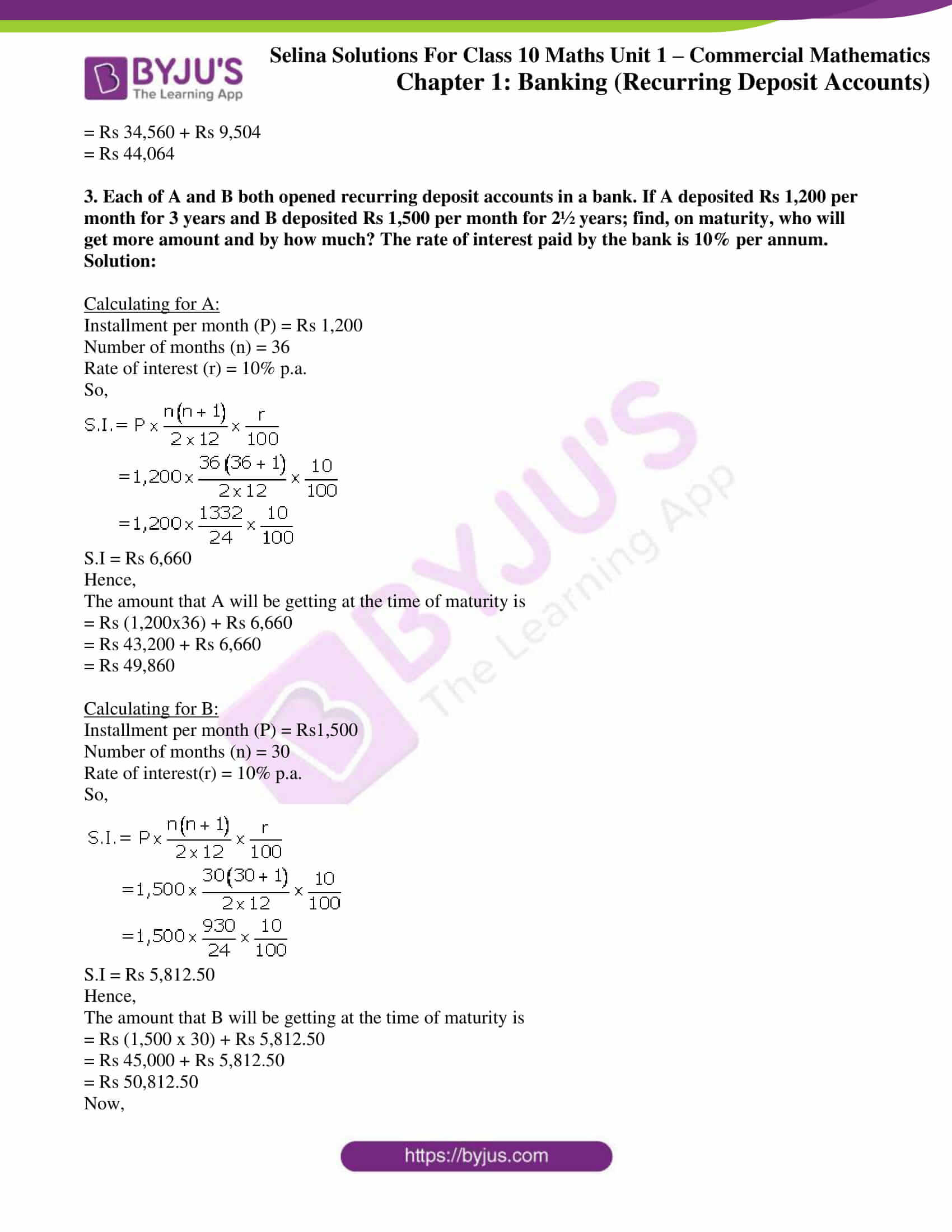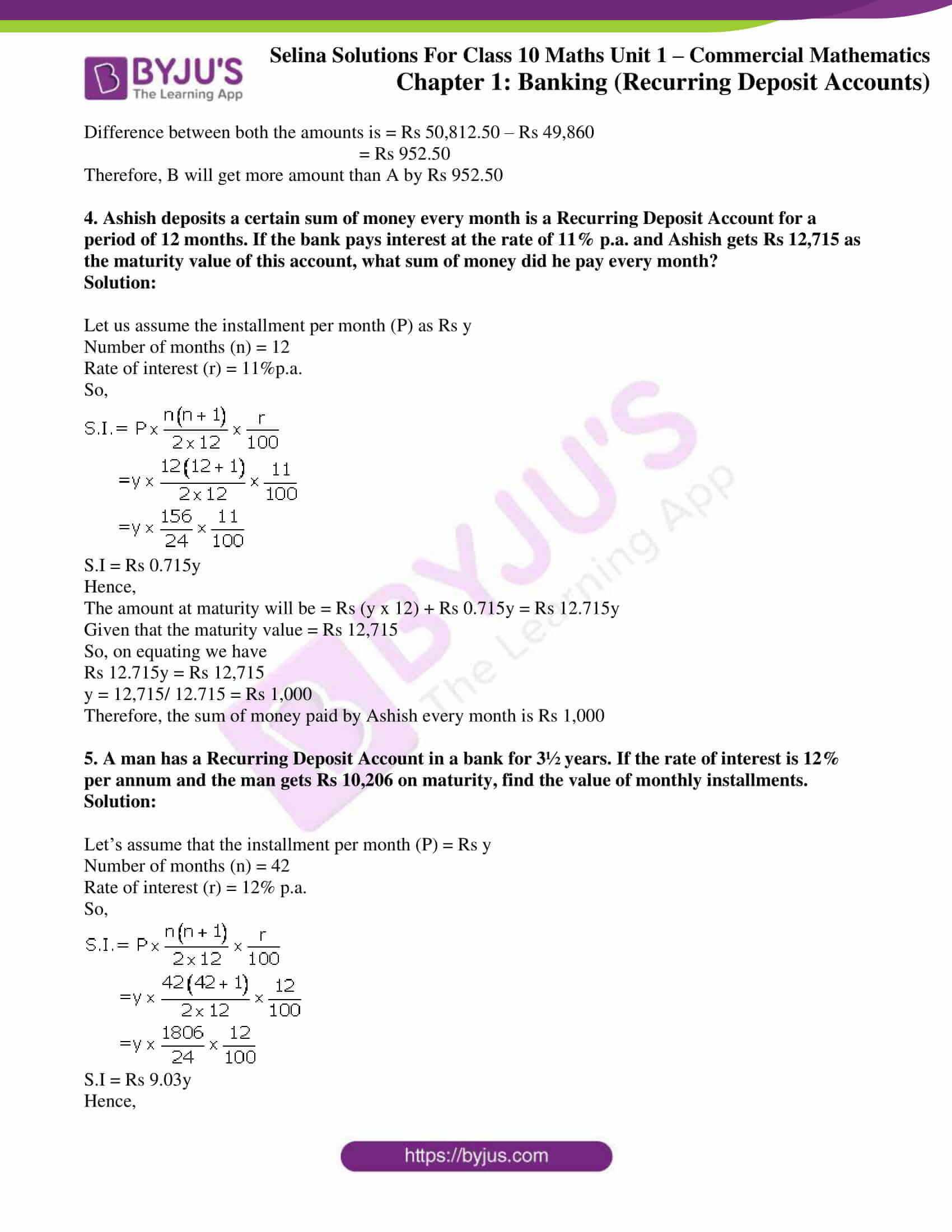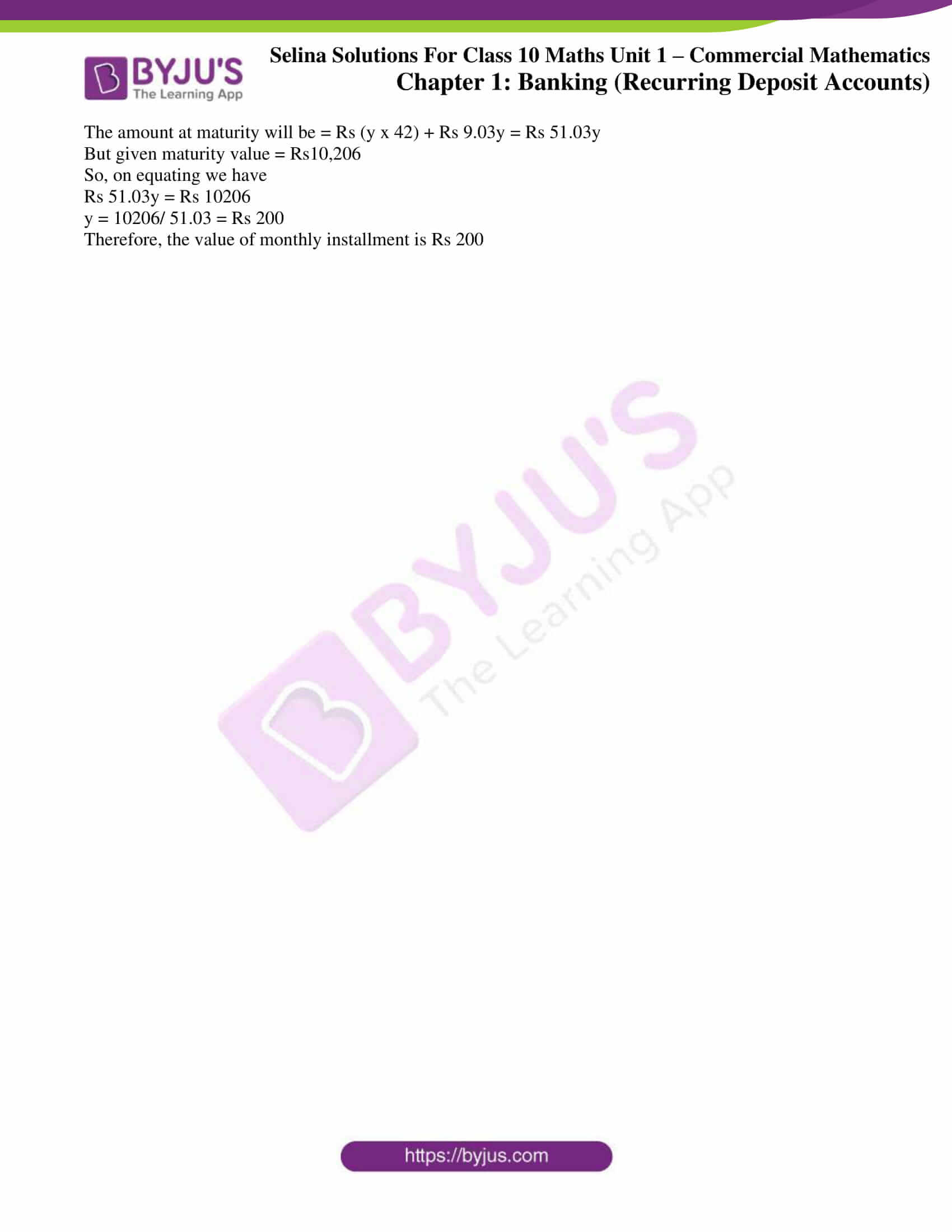### Exercise 2(A) Page No: 23

1. Manish opens a Recurring Deposit Account with the Bank of Rajasthan and deposits Rs. 600 per month for 20 months. Calculate the maturity value of this account, if the bank pays interest at the rate of 10% per annum.

Solution:

From the question, we have

Installment per month (P) = Rs 600

Number of months (n) = 20

Rate of interest(r) = 10% p.a.

So,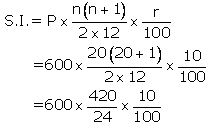S.I = Rs 1,050

Therefore,

The amount that Manish will be getting at the time of maturity is

= Rs (600 x 20) + 1,050

= Rs 12,000 + 1,050

= Rs 13,050

2. Mrs. Mathew opened a Recurring Deposit Account in a certain bank and deposited Rs. 640 per month for 41/2 years. Find the maturity value of this account, if the bank pays interest at the rate of 12% per year.

Solution:

Installment per month (P) = Rs 640

Number of months (n) = 54

Rate of interest (r) = 12% p.a.

So,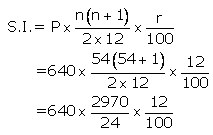S.I = Rs 9,504

Therefore,

The amount that Mrs. Mathew will be getting at the time of maturity is

= Rs (640 x 54)+ Rs 9,504

= Rs 34,560 + Rs 9,504

= Rs 44,064

3. Each of A and B both opened recurring deposit accounts in a bank. If A deposited Rs 1,200 per month for 3 years and B deposited Rs 1,500 per month for 2½ years; find, on maturity, who will get more amount and by how much? The rate of interest paid by the bank is 10% per annum.

Solution:

Calculating for A:

Installment per month (P) = Rs 1,200

Number of months (n) = 36

Rate of interest (r) = 10% p.a.

So,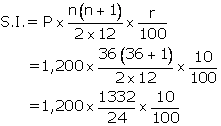S.I = Rs 6,660

Hence,

The amount that A will be getting at the time of maturity is

= Rs (1,200×36) + Rs 6,660

= Rs 43,200 + Rs 6,660

= Rs 49,860

Calculating for B:

Installment per month (P) = Rs1,500

Number of months (n) = 30

Rate of interest(r) = 10% p.a.

So,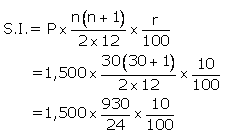S.I = Rs 5,812.50

Hence,

The amount that B will be getting at the time of maturity is

= Rs (1,500 x 30) + Rs 5,812.50

= Rs 45,000 + Rs 5,812.50

= Rs 50,812.50

Now,

Difference between both the amounts is = Rs 50,812.50 – Rs 49,860

= Rs 952.50

Therefore, B will get more amount than A by Rs 952.50

4. Ashish deposits a certain sum of money every month is a Recurring Deposit Account for a period of 12 months. If the bank pays interest at the rate of 11% p.a. and Ashish gets Rs 12,715 as the maturity value of this account, what sum of money did he pay every month?

Solution:

Let us assume the installment per month (P) as Rs y

Number of months (n) = 12

Rate of interest (r) = 11%p.a.

So,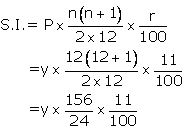S.I = Rs 0.715y

Hence,

The amount at maturity will be = Rs (y x 12) + Rs 0.715y = Rs 12.715y

Given that the maturity value = Rs 12,715

So, on equating we have

Rs 12.715y = Rs 12,715

y = 12,715/ 12.715 = Rs 1,000

Therefore, the sum of money paid by Ashish every month is Rs 1,000

5. A man has a Recurring Deposit Account in a bank for 3½ years. If the rate of interest is 12% per annum and the man gets Rs 10,206 on maturity, find the value of monthly installments.

Solution:

Let’s assume that the installment per month (P) = Rs y

Number of months (n) = 42

Rate of interest (r) = 12% p.a.

So,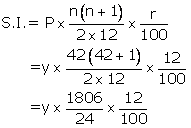S.I = Rs 9.03y

Hence,

The amount at maturity will be = Rs (y x 42) + Rs 9.03y = Rs 51.03y

But given maturity value = Rs10,206

So, on equating we have

Rs 51.03y = Rs 10206

y = 10206/ 51.03 = Rs 200

Therefore, the value of monthly installment is Rs 200

### Access another exercise of Selina Solutions Concise Maths Class 10 Chapter 2 Banking (Recurring Deposit Accounts)

Exercise 2(B) Solutions